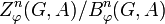# Cohomology group

This article gives a basic definition in the following area: group cohomology
View other basic definitions in group cohomology |View terms related to group cohomology |View facts related to group cohomology

## Definition

Let$G$ be a group acting on an abelian group$A$, via an action$\varphi:G \to \operatorname{Aut}(A)$. Equivalently,$A$ is a module over the (possibly non-commutative) unital group ring$\mathbb{Z}G$ of$G$ over the ring of integers.

The cohomology groups$H^n_\varphi(G,A)$ ($n = 0,1,2,3,\dots$) are abelian groups defined in the following equivalent ways.

When$\varphi$ is understood from context, the subscript${}_\varphi$ may be omitted in the notation for the cohomology group, as well as the notation for the groups of$n$-cocycles and$n$-coboundaries.

### Definition in terms of homological algebra

No. Shorthand Detailed description of$H^n_\varphi(G,A)$, the$n^{th}$ cohomology group
1 Complex based on arbitrary projective resolution Let$\mathcal{F}$ be a projective resolution for$\mathbb{Z}$ as a$\mathbb{Z}G$-module with the trivial action. Let$\mathcal{C}$ be the complex$\operatorname{Hom}_{\mathbb{Z}G}(\mathcal{F},A)$. The cohomology group$H^n_\varphi(G,A)$ is defined as the$n^{th}$ cohomology group for this complex.
2 Complex based on arbitrary injective resolution (works if category of$\mathbb{Z}G$-modules has enough injectives!) Let$\mathcal{I}$ be an injective resolution for$A$ as a$\mathbb{Z}G$-module with the specified action$\varphi$. Let$\mathcal{D}$ be the complex$\operatorname{Hom}_{\mathbb{Z}G}(\mathbb{Z},\mathcal{I})$ where$\mathbb{Z}$ has the structure of a trivial action$\mathbb{Z}G$-module. The cohomology group$H^n_\varphi(G,A)$ is defined as the$n^{th}$ cohomology group for this complex.
3 As an$\operatorname{Ext}$ functor$\operatorname{Ext}^n_{\mathbb{Z}G}(\mathbb{Z},A)$ where$\mathbb{Z}$ is a trivial$\mathbb{Z}G$-module and$A$ has the module structure specified by$\varphi$.
4 As a right derived functor$H^n_\varphi(G,A) = R^n(-^G)(A)$, i.e., it is the$n^{th}$ right derived functor of the invariants functor for$G$ (denoted$-^G$) evaluated at$A$. The invariants functor sends a$\mathbb{Z}G$-module to its submodule of elements fixed by all elements of$G$.
5 Explicit, using the bar resolution$H^n_\varphi(G,A)$, is defined as the quotient$Z^n_\varphi(G,A)/B^n_\varphi(G,A)$ where$Z^n_\varphi(G,A)$ is the group of cocycles for the action and$B^n_\varphi(G,A)$ is the group of coboundaries.
5' Explicit, using the normalized bar resolution Same as definition (5), but we use normalized cocycles and normalized coboundaries instead of arbitrary cocycles and coboundaries.

### Definition in terms of twisted cohomology

See here: Math Overflow question on the subject.

### Equivalence of definitions

Further information: Equivalence of definitions of cohomology group

The equivalence of (5) or (5') and (1) follows from the fact that (5) is the special case of (1) that arises if we choose our projective resolution as the bar resolution.

(1) and (2) are both explicit formulations of (3), based on the definition of$\operatorname{Ext}$.

The equivalence of definitions (3) and (4) follows from the fact that$A^G \cong \operatorname{Hom}_{\mathbb{Z}G}(\mathbb{Z},A)$ where$A^G$ is the set of fixed points of$A$ under$G$ and$\mathbb{Z}$ is treated as a trivial$\mathbb{Z}G$-module in the$\operatorname{Hom}$-set expression on the right side.

## Particular cases

Value of$n$ Name for$H^n_\varphi(G,A)$ Symbol for$H^n_{\varphi}(G,A)$
1 first cohomology group$H^1_\varphi(G,A)$
2 second cohomology group$H^2_\varphi(G,A)$
3 third cohomology group$H^3_\varphi(G,A)$